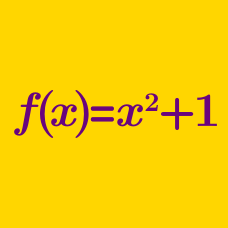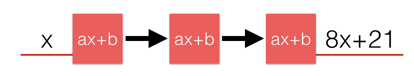Algebra

# Functions: Level 3 Challenges

Let $f : \mathbb N \to \mathbb N$ denote a function such that the table below is fulfilled.

 $x$ 1 2 3 4 5 6 7 8 9 10 11 12 $f(x)$ 8 10 12 5 2 11 9 7 1 4 3 6

Which of the following is true for integer $1\leq S \leq 12$?

Bonus: Show that for any such permutation $\sigma$, there is an $n$ such that $\sigma ^ n$ is the identity permutation.

Define a function $f : \mathbb{R} \to \mathbb{R}$ such that $f(f(x))=x^2-x+1$ for all real $x$.

Evaluate $f(0) = \, \text{? }$.

If $f(x^{2015}+1)=x^{4030}+x^{2015}+1$, then what is sum of the coefficients of $f(x^{2015}-1)?$

$\large f(x) \ f\left(\dfrac 1 x\right) = f(x) + f\left(\dfrac 1 x\right)$

A polynomial $f$ satisfies the above equation and $f(10) = 1001.$ Find the value of $f(20).$

$\large f(x)=\frac{9^x}{9^x+3}$

Suppose we define $f(x)$ as above. Let $a=f(x)+f(1-x)$ and $b=f\left(\frac1{1996}\right) + f\left(\frac2{1996}\right) + f\left(\frac3{1996}\right)+\ldots+ f\left(\frac{1995}{1996}\right).$

Evaluate $a + b$.If $f\left( x \right) =ax+b$, where $a$ and $b$ are real numbers, and $f\left( f\left( f\left( x \right) \right) \right) =8x+21$, what is $a+b$?

×

Problem Loading...

Note Loading...

Set Loading...